Next: Exercises Up: Extrema Problems and Double Previous: Background

## Examples

All of the Maple commands necessary for this lab have already been seen before.

• Find where y=cos(x) and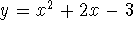intersect.
```  > solve(cos(x)=x^2+2*x-3,x);
```

```  > fsolve(cos(x)=x^2+2*x-3,x);
```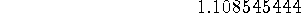```  > plot({cos(x),x^2+2*x-3},x=-5..5);
```

```  > fsolve(cos(x)=x^2+2*x-3,x=-5..0);
```These two functions intersect at approximately x=1.1085 and x=-2.7534. This can be viewed on the graph.

• Find the first order partial derivatives for the following function.Recall that there are multiple ways of doing this. These are two of the ways seen in the past.

• The first way is using the diff command.
```  > f:=(x,y)->x^2*y^2+y*sin(x);
```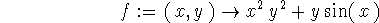```  > f_x:=diff(f(x,y),x);
```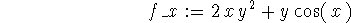```  > f_y:=diff(f(x,y),y);
```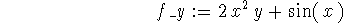• The second method is using the D command. Notice that the number in the square braces corresponds to the variable in that position when defining the function.
```  > f_x:=D(f);
``````  > f_y:=D(f);
```• Compute this integral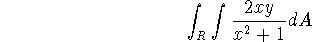over the region R where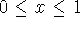and```  > inner:=int(2*x*y/(x^2+1),x=0..1);
```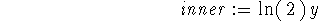```  > outer:=int(inner,y=1..3);
```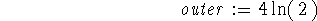```  > evalf(outer);
```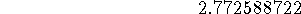The exact value of this integral is. The decimal approximation to this integral is 2.7726. Note that this integral could be computed in one step.

```  > int(int(2*x*y/(x^2+1),x=0..1),y=1..3);
```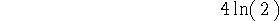• Compute the following double integral.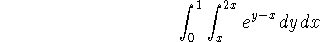```  > inner:=int(exp(y-x),y=x..2*x);
```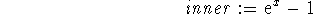```  > outer:=int(inner,x=0..1);
```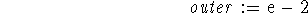```  > evalf(outer);
```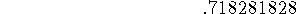The exact value of this integral is e-2. The decimal approximation to this integral is .7183. Or to save some steps, the following Maple command can be executed.

```  > int(int(exp(y-x),y=x..2*x),x=0..1);
```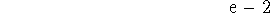Sean O Anderson
Mon Apr 21 09:58:06 EDT 1997